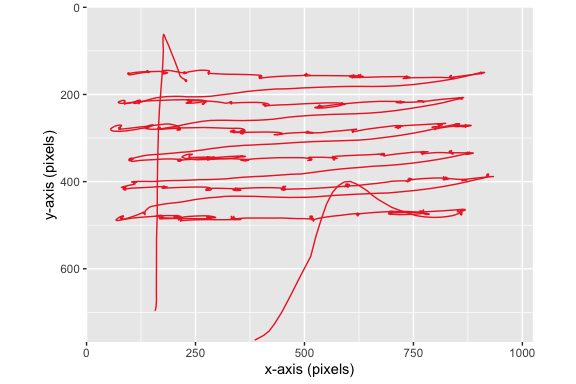#### 2021-06-03

# Import libraries required for the vignette
require(dplyr)
## Warning: package 'dplyr' was built under R version 3.6.2
require(tidyr)
## Warning: package 'tidyr' was built under R version 3.6.2
require(ggplot2)
## Warning: package 'ggplot2' was built under R version 3.6.2
require(intervals)
require(stringr)

## Converting EDFs to ASC

EyeLink eye trackers record data into binary EDFs (EyeLink Data Files), which need to be converted to plain-text ASCII (ASC) files before they can be imported with eyelinker. To convert EDFs into ASC format, you can use the EDFConverter (point-and-click) or edf2asc (command line) utilities provided in the EyeLink Developer’s Kit (downloadable on their support forums).

## Importing Data

We’ll use test data supplied by SR Research (found in the cili package for Python). The test data can be found in the extdata/ directory of the package.

# Get path of example file in package
fpath <- system.file("extdata/mono500.asc.gz", package = "eyelinker")

ASC files from longer recording sessions can be gigantic, so you can save space by compressing them using zip or gzip. To facilitate this, read.asc() supports reading compressed ASCs in .gz, .zip, .bz2, and .xz formats.

To read in all data from our example file, we call read.asc() on the filepath without any additional arguments:

dat <- read.asc(fpath)
str(dat, max.level = 1)
## List of 8
##  $raw : spec_tbl_df[,6] [1,834 × 6] (S3: spec_tbl_df/tbl_df/tbl/data.frame) ## ..- attr(*, "spec")= ## .. .. cols( ## .. .. time = col_integer(), ## .. .. xp = col_double(), ## .. .. yp = col_double(), ## .. .. ps = col_double(), ## .. .. cr.info = col_character() ## .. .. ) ##$ sacc  : tibble[,11] [8 × 11] (S3: tbl_df/tbl/data.frame)
##  $fix : tibble[,8] [12 × 8] (S3: tbl_df/tbl/data.frame) ##$ blinks: tibble[,5] [0 × 5] (S3: tbl_df/tbl/data.frame)
##  $msg : tibble[,3] [31 × 3] (S3: tbl_df/tbl/data.frame) ##$ input : tibble[,3] [4 × 3] (S3: tbl_df/tbl/data.frame)
##  $button: tibble[,4] [0 × 4] (S3: tbl_df/tbl/data.frame) ##$ info  :'data.frame':  1 obs. of  20 variables:

### Importing Events Only

For larger ASC files, especially ones recorded at a high sample rate (1000+ Hz), importing sample data can be quite slow and eat up hundreds of megabytes of memory for a single file. If you’re not interested in the raw sample data for your analysis, you can speed things up considerably by setting the samples argument to FALSE for read.asc(), meaning that it will skip parsing of raw samples for the given file:

dat_noraw <- read.asc(fpath, samples = FALSE)
str(dat_noraw, max.level = 1) # 'raw' data frame no longer present in the returned list
## List of 7
##  $sacc : tibble[,11] [8 × 11] (S3: tbl_df/tbl/data.frame) ##$ fix   : tibble[,8] [12 × 8] (S3: tbl_df/tbl/data.frame)
##  $blinks: tibble[,5] [0 × 5] (S3: tbl_df/tbl/data.frame) ##$ msg   : tibble[,3] [31 × 3] (S3: tbl_df/tbl/data.frame)
##  $input : tibble[,3] [4 × 3] (S3: tbl_df/tbl/data.frame) ##$ button: tibble[,4] [0 × 4] (S3: tbl_df/tbl/data.frame)
##  $info :'data.frame': 1 obs. of 20 variables: ### Importing Out-Of-Block Data By default, read.asc() divides samples and events into numbered blocks based on the recording “START”/“END” lines in the ASC file. However, sometimes events that occur before or after recording blocks contain important information for a task (e.g. pre-block MSG events specifying the trial stimuli, post-block input events). To retain out-of-block samples and events during import, we set the parse_all option to TRUE: dat_parseall <- read.asc(fpath, parse_all = TRUE) str(dat_parseall, max.level = 1) ## List of 8 ##$ raw   : spec_tbl_df[,6] [1,834 × 6] (S3: spec_tbl_df/tbl_df/tbl/data.frame)
##   ..- attr(*, "spec")=
##   .. .. cols(
##   .. ..   time = col_integer(),
##   .. ..   xp = col_double(),
##   .. ..   yp = col_double(),
##   .. ..   ps = col_double(),
##   .. ..   cr.info = col_character()
##   .. .. )
##  $sacc : tibble[,11] [8 × 11] (S3: tbl_df/tbl/data.frame) ##$ fix   : tibble[,8] [12 × 8] (S3: tbl_df/tbl/data.frame)
##  $blinks: tibble[,5] [0 × 5] (S3: tbl_df/tbl/data.frame) ##$ msg   : tibble[,3] [151 × 3] (S3: tbl_df/tbl/data.frame)
##  $input : tibble[,3] [16 × 3] (S3: tbl_df/tbl/data.frame) ##$ button: tibble[,4] [0 × 4] (S3: tbl_df/tbl/data.frame)
##  $info :'data.frame': 1 obs. of 20 variables: As you can see, the msg and input data frames for this file contain more events than before. For events and samples not within any block, the value of the block column will be the index of the previous block plus 0.5 (e.g. 1.5 for a message event between blocks 1 and 2). You can use block %% 1 != 0 to select all out-of-block rows in a data frame, which can be useful if you want to make out-of-block events belong to the block before or after (or exclude them from further analysis): msgs <- dat_parseall$msg
in_block <- msgs$block %% 1 == 0 # Make all out-of-block messages belong to the next block (e.g. 0.5 to 1) msgs[!in_block, ]$block <- msgs[!in_block, ]$block + 0.5 # Drop all out-of-block messages from the data msgs <- msgs[!in_block, ] ## Tracker/File Info Before looking at the actual eye movement data, let’s first take a look at the metadata for the file we just imported. The full $info table is quite large, so let’s just look at a couple useful columns. First, let’s get the tracker model, mount, and tracking mode:

dplyr::select(dat$info, c(model, mount, sample.rate, cr)) ## model mount sample.rate cr ## 1 EyeLink 1000 Plus Desktop / Monocular / Head Stabilized 500 TRUE According to the metadata, this particular file was recorded at a sample rate of 500 Hz on a EyeLink 1000 Plus tracker in the desk-mounted, head-stabilized, monocular mount configuration. This information can be helpful for writing up methods sections, as well as verifying that settings were consistent across sessions/participants for a given study. Next, we’ll look at which eyes were tracked and the reported screen resolution of the stimulus computer: dplyr::select(dat$info, c(left, right, mono, screen.x, screen.y))
##   left right mono screen.x screen.y
## 1 TRUE FALSE TRUE     1024      768

From the data, it appears that only the left eye was tracked for this file. Additionally, the resolution of the stimulus computer’s monitor was 1024x768. Finally, we’ll look at the data types for sample, event, and pupil data:

dplyr::select(dat$info, c(sample.dtype, event.dtype, pupil.dtype)) ## sample.dtype event.dtype pupil.dtype ## 1 GAZE GAZE AREA These are all the default units, but it’s important to check them in case any are a different type than you were expecting (e.g. HREF). ## Raw data The raw sample data (if present) is the largest data frame in the data, with anywhere between 125 to 2000 rows of data for every second of recording (depending on the sample rate of the tracker). For a simple non-remote monocular recording, the sample data only contains a few columns, including the time of the sample, the x/y position of the eye, and the pupil size: raw <- dat$raw
head(raw, 3)
## # A tibble: 3 x 6
##   block    time    xp    yp    ps cr.info
##   <dbl>   <int> <dbl> <dbl> <dbl> <chr>
## 1     1 7196720  513.  394.  1063 ...
## 2     1 7196722  513.  395.  1064 ...
## 3     1 7196724  514.  397   1066 ...

For a binocular recording, the raw sample data look a little different:

dat.bi <- read.asc(system.file("extdata/bino1000.asc.gz", package = "eyelinker"))

head(dat.bi$raw, 3) ## # A tibble: 3 x 9 ## block time xpl ypl psl xpr ypr psr cr.info ## <dbl> <int> <dbl> <dbl> <dbl> <dbl> <dbl> <dbl> <chr> ## 1 1 7427362 502. 411. 1103 513. 396. 1094 ..... ## 2 1 7427363 500. 412. 1103 512. 396. 1094 ..... ## 3 1 7427364 498 412. 1104 510. 394. 1094 ..... The eye variables are the same as before, but now they’re being reported for both eyes with a suffix indicating left/right (i.e. xpl is the x position of the left eye). ### Tidying up raw data It’s sometimes more convenient for plotting and analysis if the raw data are in “long” rather than “wide” format, as in the following example: raw.long <- dplyr::select(raw, time, xp, yp, block) %>% tidyr::gather("coord", "pos", xp, yp) head(raw.long, 2) ## # A tibble: 2 x 4 ## time block coord pos ## <int> <dbl> <chr> <dbl> ## 1 7196720 1 xp 513. ## 2 7196722 1 xp 513. tail(raw.long, 2) ## # A tibble: 2 x 4 ## time block coord pos ## <int> <dbl> <chr> <dbl> ## 1 7205382 4 yp 365. ## 2 7205384 4 yp 365. The eye position is now in a single column rather than two, and the column “coord” tells us if the value corresponds to the X or Y position. The benefits may not be obvious now, but it does make plotting the traces via ggplot2 a lot easier: raw.long <- mutate(raw.long, ts = (time - min(time)) / 1e3) # let's have time in sec. ggplot(raw.long, aes(ts, pos, col = coord)) + geom_point()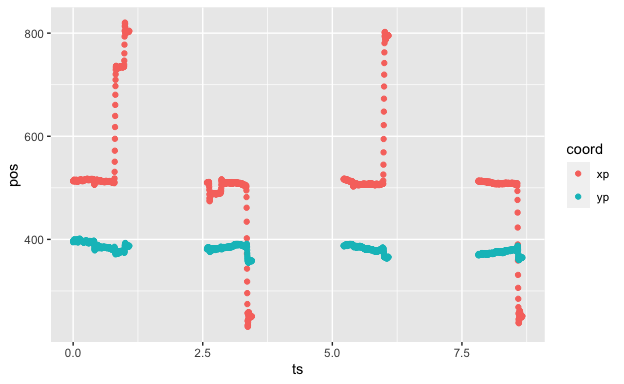In this particular file there are four separate recording periods, corresponding to different “blocks” in the ASC file, which we can check using: ggplot(raw.long, aes(ts, pos, col = coord)) + geom_line() + facet_wrap(~block)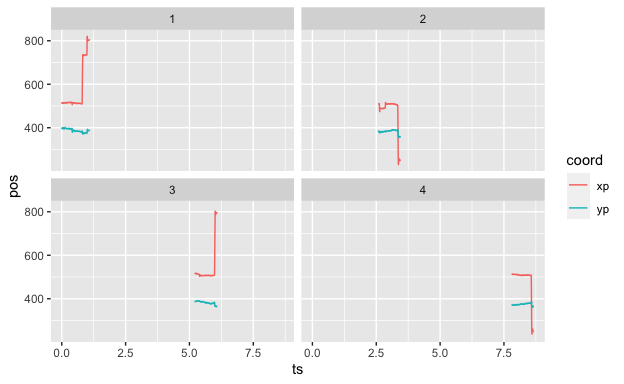### Downsampling raw data Performing operations on raw sample data can be quite slow for larger recordings (some ASC files contain several million samples), so to speed things up you can use dplyr’s filter function to downsample the data to a lower sample rate: # Downsample raw data from 500 Hz to 100 Hz by only keeping every 5th row raw_100Hz <- dplyr::filter(raw, row_number() %% 5 == 0) head(raw_100Hz, 4) ## # A tibble: 4 x 6 ## block time xp yp ps cr.info ## <dbl> <int> <dbl> <dbl> <dbl> <chr> ## 1 1 7196728 513. 398. 1062 ... ## 2 1 7196738 516. 399. 1067 ... ## 3 1 7196748 513 396 1064 ... ## 4 1 7196758 514. 395. 1062 ... ## Saccades Next, let’s look at the saccade data in our example file: sac <- dat$sac
head(sac, 3)
## # A tibble: 3 x 11
##   block   stime   etime   dur   sxp   syp   exp   eyp  ampl    pv eye
##   <dbl>   <dbl>   <dbl> <dbl> <dbl> <dbl> <dbl> <dbl> <dbl> <dbl> <fct>
## 1     1 7197124 7197134    12  514.  396.  509.  380.  0.46    57 L
## 2     1 7197510 7197546    38  511.  383   736.  373.  6.38   313 L
## 3     1 7197698 7197722    26  734.  378.  818   392.  2.37   195 L

### Labelling saccades in the raw traces

To see if the saccades have been labelled correctly, we’ll have to find the corresponding time samples in the raw data.

The easiest way to achieve this is to view the detected saccades as a set of temporal intervals, with endpoints given by stime and etime. We’ll use the %In% function to check if each time point in the raw data can be found in one of these intervals.

Sac <- cbind(sac$stime, sac$etime) # Define a set of intervals with these endpoints
raw <- mutate(raw, saccade = time %In% Sac)
head(raw, 3)
## # A tibble: 3 x 7
##   block    time    xp    yp    ps cr.info saccade
##   <dbl>   <int> <dbl> <dbl> <dbl> <chr>   <lgl>
## 1     1 7196720  513.  394.  1063 ...     FALSE
## 2     1 7196722  513.  395.  1064 ...     FALSE
## 3     1 7196724  514.  397   1066 ...     FALSE
mean(raw$saccade) * 100 # 6% of time samples correspond to saccades ##  6.161396 Now each time point labelled with “saccade == TRUE” corresponds to a saccade detected by the eye tracker. Let’s plot traces again: mutate(raw.long, saccade = time %In% Sac) %>% filter(block == 1) %>% ggplot(aes(ts, pos, group = coord, col = saccade)) + geom_line()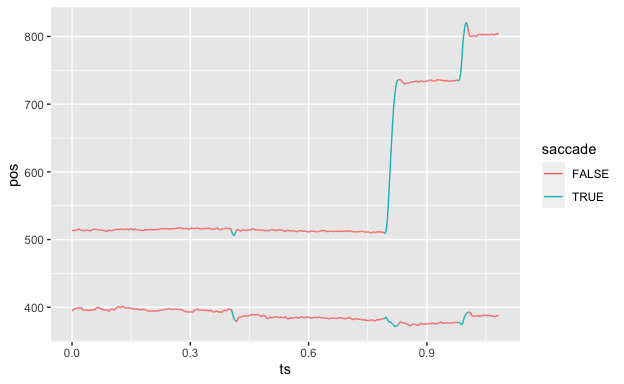## Fixations Fixations are stored in a very similar way to saccades: fix <- dat$fix
head(fix, 3)
## # A tibble: 3 x 8
##   block   stime   etime   dur   axp   ayp   aps eye
##   <dbl>   <dbl>   <dbl> <dbl> <dbl> <dbl> <dbl> <fct>
## 1     1 7196724 7197122   400  515.  396.  1050 L
## 2     1 7197136 7197508   374  513.  384.   988 L
## 3     1 7197548 7197696   150  734   376.   918 L

### Labelling fixations in the raw traces

We can re-use essentially the same code to label fixations as we did to label saccades:

Fix <- cbind(fix$stime, fix$etime) # Define a set of intervals
mutate(raw.long, fixation = time %In% Fix) %>%
filter(block == 1) %>%
ggplot(aes(ts, pos, group = coord, col = fixation)) + geom_line()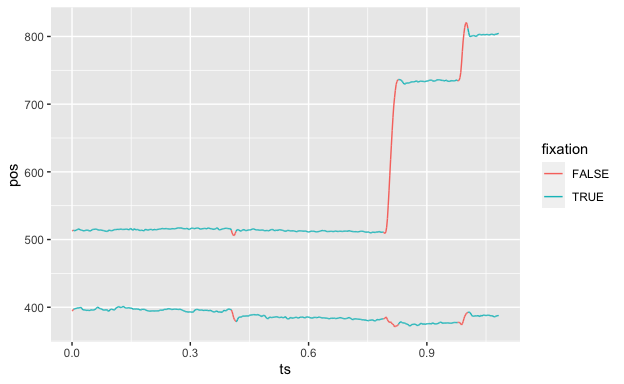We can get a fixation index using whichInterval:

head(mutate(raw, fix.index = whichInterval(time, Fix)), 4)
## # A tibble: 4 x 8
##   block    time    xp    yp    ps cr.info saccade fix.index
##   <dbl>   <int> <dbl> <dbl> <dbl> <chr>   <lgl>       <int>
## 1     1 7196720  513.  394.  1063 ...     FALSE          NA
## 2     1 7196722  513.  395.  1064 ...     FALSE          NA
## 3     1 7196724  514.  397   1066 ...     FALSE           1
## 4     1 7196726  513.  398.  1064 ...     FALSE           1

Let’s check that the average x and y positions are correct:

raw <- mutate(raw, fix.index = whichInterval(time, Fix))
fix.check <- filter(raw, !is.na(fix.index)) %>%
group_by(fix.index) %>%
summarise(axp = mean(xp), ayp = mean(yp)) %>%
ungroup
head(fix.check, 3)
## # A tibble: 3 x 3
##   fix.index   axp   ayp
##       <int> <dbl> <dbl>
## 1         1  515.  396.
## 2         2  513.  384.
## 3         3  734.  376.

We grouped all time samples according to fixation index, and computed mean x and y positions.

We verify that we recovered the right values:

all.equal(fix.check$axp, fix$axp)
##  "Mean relative difference: 4.48531e-05"
all.equal(fix.check$ayp, fix$ayp)
##  "Mean relative difference: 7.397594e-05"

## Messages

Another type of data contained in ASC files are message events, which are stored in the $msg data frame: head(dat$msg)
## # A tibble: 6 x 3
##   block     time text
##   <dbl>    <dbl> <chr>
## 1     1 12134177 -8 SYNCTIME
## 2     1 12134177 -8 !V DRAW_LIST ../../runtime/dataviewer/js/graphics/VC_1…
## 3     1 12134177 -7 !V IAREA FILE ../../runtime/dataviewer/js/aoi/IA_1.ias
## 4     1 12152026 -8 blank_screen
## 5     2 12153648 -3 SYNCTIME
## 6     2 12153648 -2 !V DRAW_LIST ../../runtime/dataviewer/js/graphics/VC_2…

The lines correspond to “MSG” lines in the imported ASC file. Since these messages can be anything, read.asc() leaves them unparsed: if you’re interested in subsetting them or extracting data from them, you can parse them yourself using packages such as stringr or with the built-in grep/grepl functions.

To illustrate, we’ll use stringr’s str_detect() function to select only rows containing the string “blank_screen”:

filter(dat$msg, str_detect(text, "blank_screen")) ## # A tibble: 4 x 3 ## block time text ## <dbl> <dbl> <chr> ## 1 1 12152026 -8 blank_screen ## 2 2 12175944 -5 blank_screen ## 3 3 12198433 -15 blank_screen ## 4 4 12223186 -10 blank_screen ## Plotting Fixation & Saccade Data What if we want to look at the pattern of fixations or saccades on a given block? We can accomplish this with ggplot2 using a couple tweaks. Importantly, we’ll need to reverse the y-axis using scale_y_reverse() since the coordinates (0,0) correspond to the top-left of the screen in the data and not the bottom-left. Additionally, we’ll want to set the scales of the X and Y axes to correspond to the screen resolution specified by screen.x and screen.y in the $info table (1024 x 768, in this case), and optionally set coord_fixed() to ensure the aspect ratio of the plot doesn’t get stretched.

For this example, we’ll plot saccades with geom_segment() and fixations using geom_point(). Additionally, we’ll make the size of the fixation points vary based on the duration of the fixation by setting size = dur in the aes() settings for geom_point():

# Get fixations/saccades for just first block
fix_b1 <- subset(dat$fix, block == 1) sacc_b1 <- subset(dat$sacc, block == 1)

# Actually plot fixations and saccades
ggplot() +
geom_segment(data = sacc_b1,
aes(x = sxp, y = syp, xend = exp, yend = eyp),
arrow = arrow(), size = 1, alpha = 0.5, color = "grey40"
) +
geom_point(data = fix_b1,
aes(x = axp, y = ayp, size = dur, color = eye),
alpha = 0.5, color = "blue"
) +
scale_x_continuous(expand = c(0, 0), limits = c(0, dat$info$screen.x)) +
scale_y_reverse(expand = c(0, 0), limits = c(dat$info$screen.y, 0)) +
labs(x = "x-axis (pixels)", y = "y-axis (pixels)") +
coord_fixed() # Keeps aspect ratio from getting distorted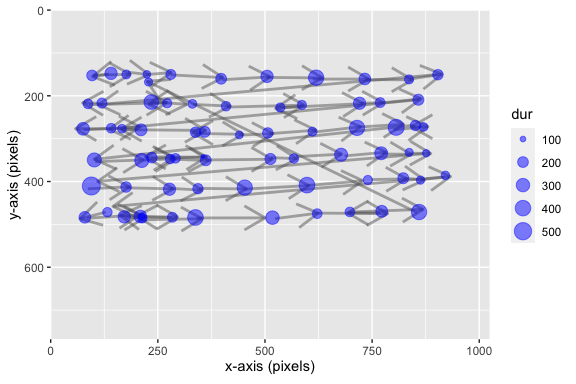Similarly, raw gaze samples can be plotted out using geom_path():

raw_b1 <- subset(dat$raw, block == 1) ggplot() + geom_path(data = raw_b1, aes(x = xp, y = yp), size = 0.5, color = "firebrick2") + scale_x_continuous(expand = c(0, 0), limits = c(0, dat$info$screen.x)) + scale_y_reverse(expand = c(0, 0), limits = c(dat$info\$screen.y, 0)) +
labs(x = "x-axis (pixels)", y = "y-axis (pixels)") +
coord_fixed() # Keeps aspect ratio from getting distorted